快速_快速排序 - CSDN

• 快速排序算法介绍 划分问题：把数组的各个元素重排后分成左右两个部分，使得左边任意元素都小于或等于右边任意元素。 递归求解：把左右两部分分别排序。 快速排序代码 #include <iostream> using namespace ...
快速排序算法介绍
划分问题：把数组的各个元素重排后分成左右两个部分，使得左边任意元素都小于或等于右边任意元素。
递归求解：把左右两部分分别排序。
快速排序代码
#include <iostream>
using namespace std;
void swap(int & a,int & b)
{//交换变量a，b值
int tmp=a;
a=b;
b=tmp;
}
void QuickSort(int a[],int s,int e)
{
if(s>=e)
return ;
int k=a[s];
int i=s,j=e;
while(i!=j)
{
while(j>i&&a[j]>=k) --j;
swap(a[i],a[j]);
while(i<j&&a[i]<=k) ++i;
swap(a[i],a[j]);
}//处理完后，a[i]=k
QuickSort(a,s,i-1);
QuickSort(a,i+1,e);
}
int a[]={93,27,30,2,8,12,2,8,30,89};
int main ()
{
int size=sizeof(a)/sizeof(int);
QuickSort(a,0,size-1);
for(int i=0;i<size;++i)
{
cout<<a[i]<<",";
}
cout<<endl;
return 0;
}


快速选择问题
输入n个整数和一个正整数k（1<=k<=n），输出这些整数从小到大排序后的第k个，n<=107。
分析
假设在快速排序的“划分”结束后，数组A[p……r]被分成了A[p……q]和A[q+1……r]，则可以根据左边的元素个数q-p+1和k的大小关系只在左边或者只在右边递归求解。
代码
#include <iostream>
#include <algorithm>

using namespace std;

int qsort(int *a, int left, int right, int k) {//快速排序算法
if (left > right) return 0;       //递归边界
int centerV = a[left + (right - left) / 2];      //取标兵值

int i = left, j = right;
while (i <= j) {
while (i <= j) {//从左往右扫描大于标兵值的元素，放在标兵值右侧
if (a[i] >= centerV) break;
i++;
}
while (j >= i) {//从右往左扫描小于标兵值的元素，放在标兵值左侧
if (a[j] <= centerV) break;
j--;
}
if (i > j) break;     //退出条件
swap(a[i], a[j]);
i++;
j--;
}
if (k - 1 <= i)     //递归求左半解
return qsort(a, left, j, k);
else if (k - 1 > i + 1)       //递归求右半解
return qsort(a, i, right, k);
else
return a[k - 1];
}

int QuickSort(int *pInt, int n, int k) {
return qsort(pInt, 0, n - 1, k);
}

int main() {
int a = {1, 2, 3, 4, 9, 33, 12, 8, 9, 10};
int n = 10, k = 9;
cout << "Before sorting: ";
for (int i = 0; i < n; ++i) {
cout << a[i] << ' ';
}
cout << endl;
int ans = QuickSort(a, n, k);
cout << "After sorting: ";
for (int i = 0; i < n; ++i) {
cout << a[i] << ' ';
}
cout << endl;
cout << "Number " << k << " is: " << ans << endl;
return 0;
}



展开全文• ## 快速排序---(面试碰到过好几次)

万次阅读 多人点赞 2020-07-09 20:32:13
快速排序,说白了就是给基准数据找其正确索引位置的过程.    如下图所示,假设最开始的基准数据为数组第一个元素23,则首先用一个临时变量去存储基准数据,即tmp=23;然后分别从数组的两端扫描数组，设两个指示...
原理:
快速排序,说白了就是给基准数据找其正确索引位置的过程.
如下图所示,假设最开始的基准数据为数组第一个元素23,则首先用一个临时变量去存储基准数据,即tmp=23;然后分别从数组的两端扫描数组，设两个指示标志:low指向起始位置，high指向末尾.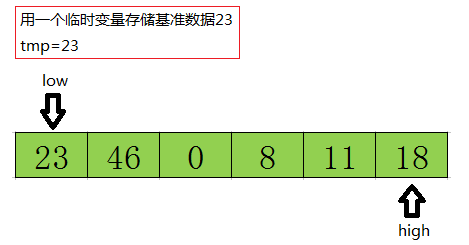首先从后半部分开始，如果扫描到的值大于基准数据就让high减1,如果发现有元素比该基准数据的值小(如上图中18<=tmp)，就将high位置的值赋值给low位置 ,结果如下: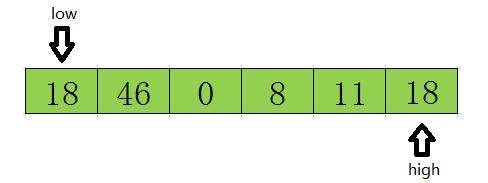然后开始从前往后扫描,如果扫描到的值小于基准数据就让low加1,如果发现有元素大于基准数据的值(如上图46=>tmp)，就再将low位置的值赋值给high位置的值,指针移动并且数据交换后的结果如下: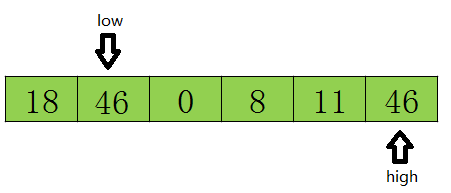然后再开始从后向前扫描,原理同上,发现上图11<=tmp,则将low位置的值赋值给high位置的值 ,结果如下:
然后再开始从后向前扫描,原理同上,发现上图11<=tmp,则将high位置的值赋值给low位置的值,结果如下: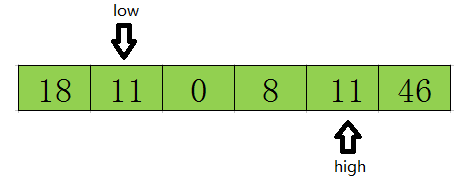然后再开始从前往后遍历,直到low=high结束循环,此时low或high的下标就是基准数据23在该数组中的正确索引位置.如下图所示.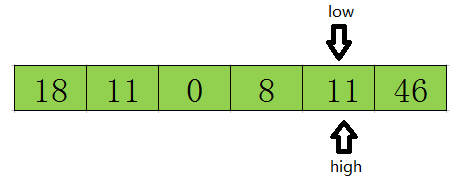这样一遍走下来,可以很清楚的知道,其实快速排序的本质就是把基准数大的都放在基准数的右边,把比基准数小的放在基准数的左边,这样就找到了该数据在数组中的正确位置.
以后采用递归的方式分别对前半部分和后半部分排序，当前半部分和后半部分均有序时该数组就自然有序了。
一些小结论
从上面的过程中可以看到:
①先从队尾开始向前扫描且当low < high时,如果a[high] > tmp,则high–,但如果a[high] < tmp,则将high的值赋值给low,即arr[low] = a[high],同时要转换数组扫描的方式,即需要从队首开始向队尾进行扫描了
②同理,当从队首开始向队尾进行扫描时,如果a[low] < tmp,则low++,但如果a[low] > tmp了,则就需要将low位置的值赋值给high位置,即arr[low]  = arr[high],同时将数组扫描方式换为由队尾向队首进行扫描.
③不断重复①和②,知道low>=high时(其实是low=high),low或high的位置就是该基准数据在数组中的正确索引位置.
按照上诉理论我写的代码如下:
package com.nrsc.sort;

public class QuickSort {
public static void main(String[] args) {
int[] arr = { 49, 38, 65, 97, 23, 22, 76, 1, 5, 8, 2, 0, -1, 22 };
quickSort(arr, 0, arr.length - 1);
System.out.println("排序后:");
for (int i : arr) {
System.out.println(i);
}
}

private static void quickSort(int[] arr, int low, int high) {

if (low < high) {
// 找寻基准数据的正确索引
int index = getIndex(arr, low, high);

// 进行迭代对index之前和之后的数组进行相同的操作使整个数组变成有序
//quickSort(arr, 0, index - 1); 之前的版本，这种姿势有很大的性能问题，谢谢大家的建议
quickSort(arr, low, index - 1);
quickSort(arr, index + 1, high);
}

}

private static int getIndex(int[] arr, int low, int high) {
// 基准数据
int tmp = arr[low];
while (low < high) {
// 当队尾的元素大于等于基准数据时,向前挪动high指针
while (low < high && arr[high] >= tmp) {
high--;
}
// 如果队尾元素小于tmp了,需要将其赋值给low
arr[low] = arr[high];
// 当队首元素小于等于tmp时,向前挪动low指针
while (low < high && arr[low] <= tmp) {
low++;
}
// 当队首元素大于tmp时,需要将其赋值给high
arr[high] = arr[low];

}
// 跳出循环时low和high相等,此时的low或high就是tmp的正确索引位置
// 由原理部分可以很清楚的知道low位置的值并不是tmp,所以需要将tmp赋值给arr[low]
arr[low] = tmp;
return low; // 返回tmp的正确位置
}
}




展开全文• 看代码： public static int[] partition(int[] arr,int i,int T) { int lt = i-1,gt = T; while (i<gt) { if(arr[i]==arr[T]) { ++i; } ...
看代码：

public static int[] partition(int[] arr,int i,int T)
{

int lt = i-1,gt = T;
while (i<gt) {
if(arr[i]==arr[T]) {
++i;
}
else if(arr[i]<arr[T]) {
swap(arr,++lt,i++);
}else{
swap(arr,--gt,i);
}
}
swap(arr,gt,T);
return new int[]{lt,gt+1};//lt==gt ?

}

public static void swap(int[] arr,int l,int r)
{
if(arr[l]==arr[r])return;
arr[l]^=arr[r];
arr[r]^=arr[l];
arr[l]^=arr[r];
}

public static void quickSort(int[] arr,int l,int r) {
if(r<=l) return;
swap(arr,l+(int)Math.random()*(r-l+1),r);
int[] eq = partition(arr,l,r);
quickSort(arr,l,eq);
quickSort(arr,eq,r);

}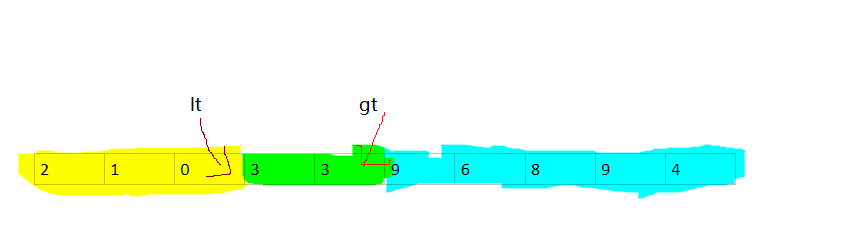如图所示：
3路快速排序,
这一轮选择的基准点是 3
黄色的表示小于3的区域，蓝色的表示大于3的区域，绿色的表示等于3的区域
partition函数返回 lt,和gt+1 这个下标，即 在【lt+1,gt】这个区间里面
都是相等的元素，一定是有序的，剩下的就是排这个区间外的其他元素
排序选择的标定点最好与数据原本的顺序无关，使得标定点左右两边的区域的长度相等
这里我在 给定的 [l,r] 区间里面随机选择一个元素与 r位置的交换，也就是说标定点位置设为 r位置，我把这个位置用 T 表示
举个例子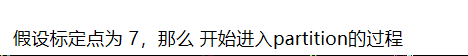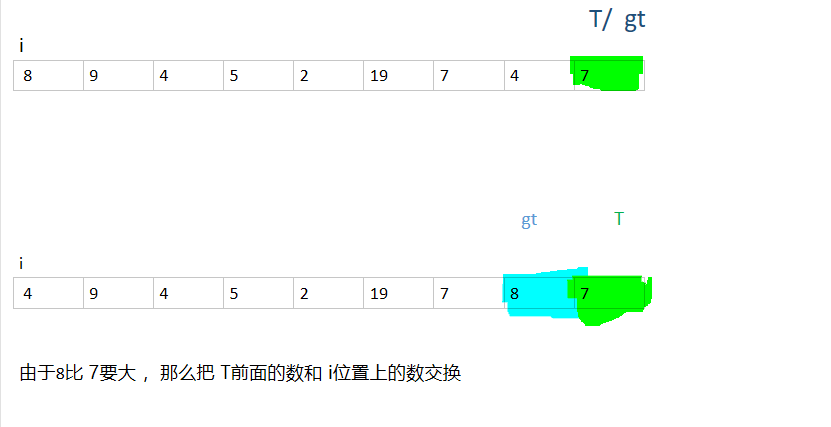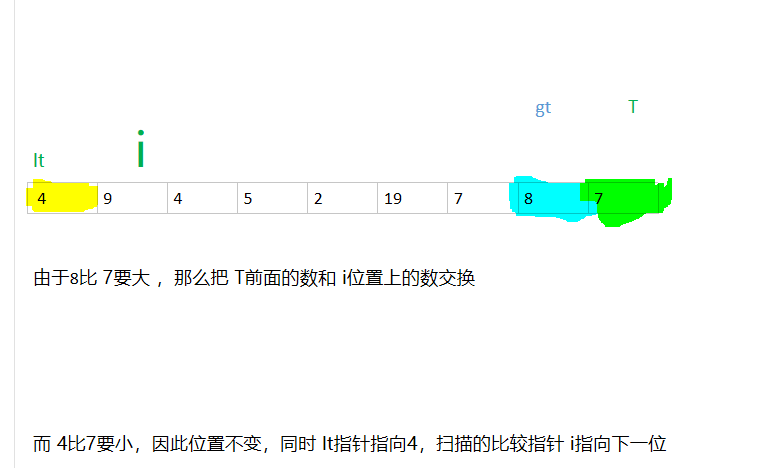lt部分推着 i走，当扫描指针i到达 gt位置的时候说明空白部分的长度为0，也就是说 这一轮的partiton 完成了这时，把 gt指向的位置和 T所在的位置元素交换把 lt位置和 gt+1位置返回，这就是下一轮要排序的范围


展开全文• 题目: 2019银川网络赛L：Continuous Intervals 题意： 定义一个连续区间：如果将排序后，相邻元素的差的绝对值不大于，则称其为一个连续区间，现在给定包含个元素的数组，求有多少个连续区间 ...
题目:

2019银川网络赛L：Continuous Intervals

题意：

定义一个连续区间：如果将  排序后，相邻元素的差的绝对值不大于 ，则称其为一个连续区间，现在给定包含 个元素的数组，求有多少个连续区间

分析：

虽然网络选拔赛直接拿原题有点恶心，但题还是好题

如果一个区间  符合连续区间的定义，设这个区间内的最大值为 ，最小值为 ，不同元素的个数为 ，那么会有：，这类统计区间个数的问题，需要套路的枚举一个端点，然后统计有多少个另一个端点符合条件，枚举左端点相当于删除数，枚举右端点相当于添加数；转化一下公式：，而对于任意区间有如下推论：，这意味着我们只需要维护区间中  的最小值及其个数，就可以快速得到另一个端点的数量；添加数容易维护区间最值，所以考虑枚举右端点 ，每次添加一个数(移动右端点)，我们需要知道这个数作为最大值能影响的最远左端点 ，这个可以通过单调栈得到，那么我们就需要修改  的最大值；如果直接暴力修改，复杂度太高，考虑在  的值上再加上即将修改的最大值和原有最大值的差值就行了，所以还得记录每个最大值影响的区间，最小值同理，只需要用  记录每个数上次出现的位置就可维护

代码：

#include <bits/stdc++.h>

#define fi first
#define se second
#define pii pair<int,int>
#define PII pair<int,pii>
#define sz(x) (int)(x).size()
using namespace std;
typedef long long LL;
const int maxn = 1e5+16;
int T,n,m,a[maxn];
map<int,int> mp;
stack<PII> s1,s2;          //PII.fi代表数值,PII.se代表这个数值影响的区间
LL lazy[maxn<<2],MIN[maxn<<2],cnt[maxn<<2];
inline void pushdown(int x){
MIN[x<<1] += lazy[x]; MIN[x<<1|1] += lazy[x];
lazy[x<<1] += lazy[x]; lazy[x<<1|1] += lazy[x];
lazy[x] = 0;
}
void BuildTree(int l,int r,int x){
cnt[x] = MIN[x] = lazy[x] = 0;
if(l == r){
cnt[x] = 1;
return ;
}
int mid = (l+r) >> 1;
BuildTree(l,mid,x<<1);
BuildTree(mid+1,r,x<<1|1);
}
void UpdataTree1(int l,int r,int L,int R,int x,LL val){
if(l > R || r < L) return;
if(l >= L && r <= R){
MIN[x] += val;
lazy[x] += val;
return ;
}
if(lazy[x]) pushdown(x);
int mid = (l+r)>>1;
UpdataTree1(l,mid,L,R,x<<1,val);
UpdataTree1(mid+1,r,L,R,x<<1|1,val);
MIN[x] = min(MIN[x<<1],MIN[x<<1|1]); cnt[x] = 0;
if(MIN[x] == MIN[x<<1]) cnt[x] += cnt[x<<1];
if(MIN[x] == MIN[x<<1|1]) cnt[x] += cnt[x<<1|1];
}
void QueryTree(int l,int r,int L,int R,int x,LL &res){
if(l > R || r < L) return ;
if(l >= L && r <= R){
if(MIN[x] == -1) res += cnt[x];
return ;
}
if(lazy[x]) pushdown(x);
int mid = (l+r) >> 1;
QueryTree(l,mid,L,R,x<<1,res);
QueryTree(mid+1,r,L,R,x<<1|1,res);
}
LL solve(){
mp.clear(); LL res = 0;
while(!s1.empty()) s1.pop();
while(!s2.empty()) s2.pop();
s1.push(PII(2e9,pii(0,0))); s2.push(PII(0,pii(0,0)));
for(int i = 1;i <= n; ++i){
UpdataTree1(1,n,i,i,1,-1);
if(!mp.count(a[i])) UpdataTree1(1,n,1,i-1,1,-1);
else UpdataTree1(1,n,mp[a[i]]+1,i-1,1,-1);
int last = i;
while(!s1.empty()&&s1.top().fi<a[i]){
UpdataTree1(1,n,s1.top().se.fi,last-1,1,a[i]-s1.top().fi);
last = s1.top().se.fi; s1.pop();
}
last = i;
while(!s2.empty()&&s2.top().fi>a[i]){
UpdataTree1(1,n,s2.top().se.fi,last-1,1,s2.top().fi-a[i]);
last = s2.top().se.fi; s2.pop();
}
mp[a[i]] = i; s1.push(PII(a[i],pii(s1.top().se.se+1,i)));s2.push(PII(a[i],pii(s2.top().se.se+1,i)));
QueryTree(1,n,1,i,1,res);
}
return res;
}
int main(){
int T; cin >> T;
for(int Case = 1;Case <= T; ++Case){
scanf("%d",&n); BuildTree(1,n,1);
for(int i = 1;i <= n; ++i) scanf("%d",a+i);
printf("Case #%d: %lld\n",Case,solve());
}
return 0;
}


展开全文线段树
• 快速排序由于排序效率在同为O(N*logN)的几种排序方法中效率较高，因此经常被采用，再加上快速排序思想----分治法也确实实用，因此很多软件公司的笔试面试，包括像腾讯，微软等知名IT公司都喜欢考这个，还有大大小的...微软 算法 腾讯
• 插入排序以及选择排序请查阅我往期博客：http://blog.csdn.net/sayhello_world/article/details/61927082 冒泡排序： 思想：两两交换，大的放到后面。重复size-1次 代码实现： ...//冒泡排序面试题 排序 冒泡排序 快速排序
• 1.最简单排序实现 冒泡排序的思想其实很简单，它是一种简单的选择排序，在细节上有很多种优化的方法。 它的基本思想是：两两比较相邻记录的关键字，如果反序则进行交换。 首先先来看一段简单的冒泡排序：//冒泡...冒泡排序
• C语言排序实例（选择、冒泡、插入、折半、快速）： void select_sort(int *a, int n); //选择法排序 void bubble_sort(int *a, int n); //冒泡法排序 void insert_sort(int *a, int n); //插入法排序 void shell_...c语言 排序
• 原文链接https://blog.csdn.net/foreverling/article/details/43798223, 感谢原文作者 楚兴大牛的分享,转载只为了能方便阅读,如有侵权还请联系,我将马上将文章删除 九大排序算法总结 &amp;...a算法 冒泡 快速 归并 java
• 四大排序的时间复杂度和空间复杂度时间复杂度时间复杂度的表示方法时间复杂度的分析和计算方法常见的几种时间复杂度常见的时间复杂度排序空间复杂度 时间复杂度 (1)时间频率 一个算法执行所耗费的时间，从理论上是不...
• ## 快速排序算法——C/C++

万次阅读 多人点赞 2020-06-25 10:48:09
快速排序 1、算法思想 快速排序的基本思想：通过一趟排序将待排记录分隔成独立的两部分，其中一部分记录的关键字均比另一部分的关键字小，则可分别对这两部分记录继续进行排序，以达到整个序列有序。 2、实现原理 ...
• ## 快速排序（过程图解）

万次阅读 多人点赞 2018-12-10 14:58:45
假设我们现在对“6 1 2 7 9 3 4 5 10 8”这个10个数进行排序。首先在这个序列中随便找一个数作为基准数（不要被这个名词吓到了，就是一个用来参照的数，待会你就知道它用来做啥的了）。...
• ## 快速幂

万次阅读 多人点赞 2019-12-09 20:21:24
以前我看快速幂真的看不懂，但是当我慢慢对递归（递归大法好）有一些理解后，对二分又理解一些后，就能够自己写出快速幂的递归写法了。 求 a^b % m的值，这个用普通算法我就不说了，时间复杂度O（b） 当我知道快速...
• ## 算法 - 快速求一个整数的7倍

万次阅读 多人点赞 2019-03-13 22:40:24
分享一个大牛的人工智能教程。零基础！...乘法运算相对比较慢，所以快速的方法就是将这个乘法转换成加减法和移位操作。 可以将此整数先左移三位（*8）然后再减去原值：X &lt;&lt; 3 – X。 ...算法
• 记录时间2019年5月18日 [下载地址] 登陆百度账号，下载分享链接;现在不登陆账号没办法下载； 出现问题就解决问题： ...遇到403(22)错误： 被百度云盘限速了，方法：换网，换IP； ...
• 1: 快速到最后一行： shift + G 2:快速到第一行： 1 + shift +G 3: 快速到第40 行： 40 + shift + G
• 输入syso然后ALT+/ 就可以了 或者首先输入sysout，然后ALT+/
• ## Z平台-开源免费的JAVA快速开发平台

万次阅读 多人点赞 2020-06-11 10:12:46
Z平台是开源免费的JAVA快速开发平台，通过Z平台集成开发环境，以零编码、动态配置的方式能够快速开发BS管理系统。同时该平台还可以做为APP、微信、各种小程序等项目的服务端来使用，为前端项目提供数据接口。并且Z...快速开发平台 后台系统 java
• 解读PMP考点：快速跟进和赶工的区别 赶工和快速跟进都是进度压缩技术。 进度压缩技术是指在不缩减项目范围的前提下，通过缩短或加快进度工期，以满足进度制约因素、强制日期或者其他进度目标。 赶工和进度压缩的...
• ## 用C语言实现快速排序算法

万次阅读 多人点赞 2019-08-13 14:39:59
一、快速排序算法（Quicksort） 1. 定义 快速排序由C. A. R. Hoare在1962年提出。快速排序是对冒泡排序的一种改进，采用了一种分治的策略。 2. 基本思想 通过一趟排序将要排序的数据分割成独立的两部分，其中...排序 快速排序算法 C实现快速排序
...